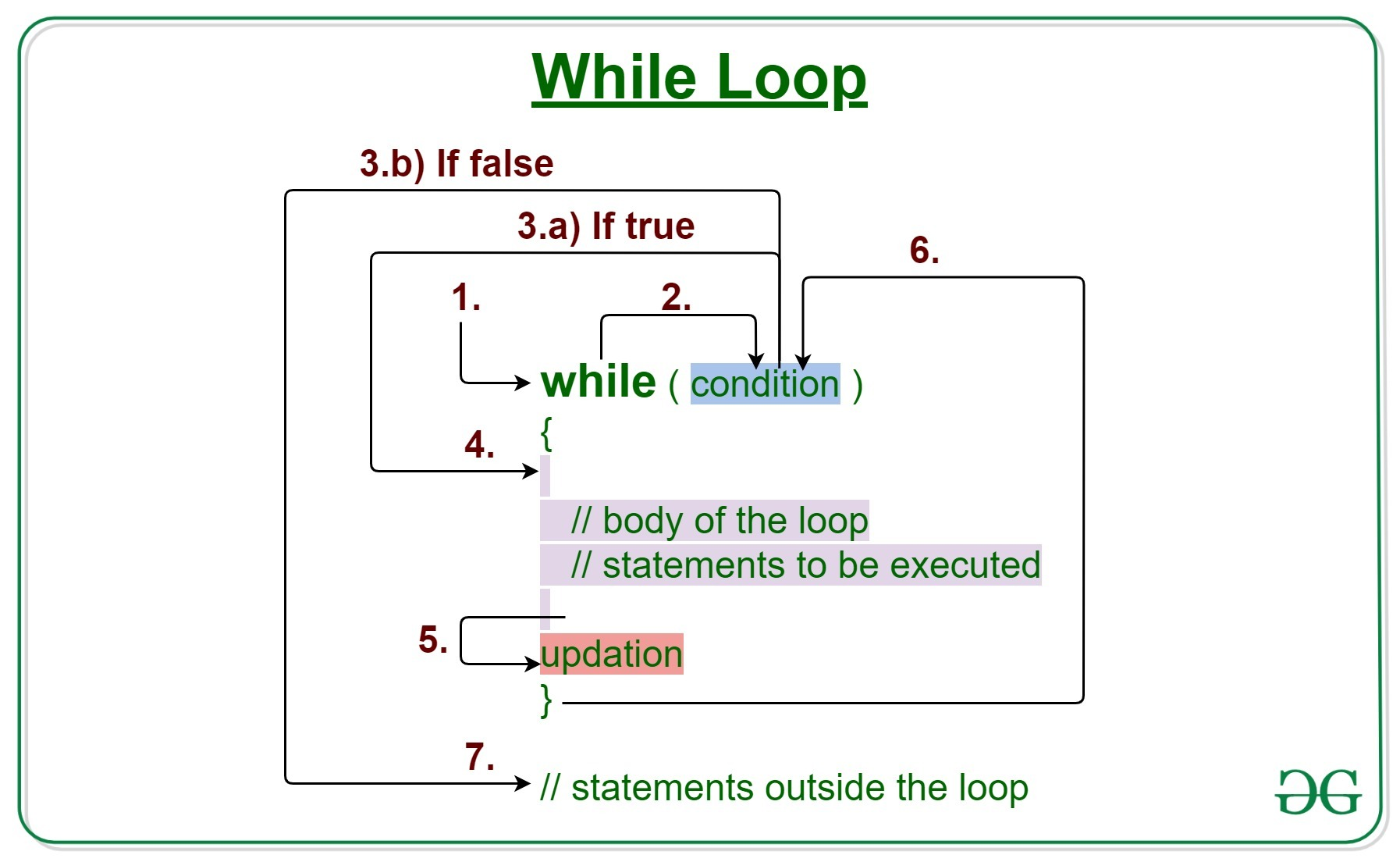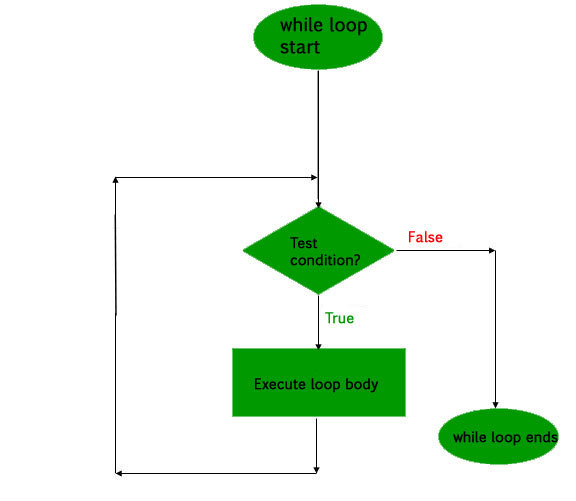# C/C++ while loop with Examples

Loops in C/C++ come into use when we need to repeatedly execute a block of statements.

During the study of ‘for’ loop in C or C++, we have seen that the number of iterations is known beforehand, i.e. the number of times the loop body is needed to be executed is known to us. The while loop in C/C++ is used in situations where we do not know the exact number of iterations of loop beforehand. The loop execution is terminated on the basis of the test condition.Syntax:

```while (test_expression)
{
// statements

update_expression;
}```

The various parts of the While loop are:

1. Test Expression: In this expression we have to test the condition. If the condition evaluates to true then we will execute the body of the loop and go to update expression. Otherwise, we will exit from the while loop.
Example:

`i <= 10`
2. Update Expression: After executing the loop body, this expression increments/decrements the loop variable by some value.
Example:

`i++;`

How does a While loop executes?

1. Control falls into the while loop.
2. The flow jumps to Condition
3. Condition is tested.
1. If Condition yields true, the flow goes into the Body.
2. If Condition yields false, the flow goes outside the loop
4. The statements inside the body of the loop get executed.
5. Updation takes place.
6. Control flows back to Step 2.
7. The do-while loop has ended and the flow has gone outside.

Flowchart while loop (for Control Flow):Example 1: This program will try to print “Hello World” 5 times.

## C

 `// C program to illustrate while loop ` ` `  `#include ` ` `  `int` `main() ` `{ ` `    ``// initialization expression ` `    ``int` `i = 1; ` ` `  `    ``// test expression ` `    ``while` `(i < 6) { ` `        ``printf``(``"Hello World\n"``); ` ` `  `        ``// update expression ` `        ``i++; ` `    ``} ` ` `  `    ``return` `0; ` `} `

## C++

 `// C++ program to illustrate while loop ` ` `  `#include ` `using` `namespace` `std; ` ` `  `int` `main() ` `{ ` `    ``// initialization expression ` `    ``int` `i = 1; ` ` `  `    ``// test expression ` `    ``while` `(i < 6) { ` `        ``cout << ``"Hello World\n"``; ` ` `  `        ``// update expression ` `        ``i++; ` `    ``} ` ` `  `    ``return` `0; ` `} `

Output:

```Hello World
Hello World
Hello World
Hello World
Hello World
```

Dry-Running Example 1: The program will execute in the following manner.

```1. Program starts.
2. i is initialized with value 1.
3. Condition is checked. 1 < 6 yields true.
3.a) "Hello World" gets printed 1st time.
3.b) Updation is done. Now i = 2.
4. Condition is checked. 2 < 6 yields true.
4.a) "Hello World" gets printed 2nd time.
4.b) Updation is done. Now i = 3.
5. Condition is checked. 3 < 6 yields true.
5.a) "Hello World" gets printed 3rd time
5.b) Updation is done. Now i = 4.
6. Condition is checked. 4 < 6 yields true.
6.a) "Hello World" gets printed 4th time
6.b) Updation is done. Now i = 5.
7. Condition is checked. 5 < 6 yields true.
7.a) "Hello World" gets printed 5th time
7.b) Updation is done. Now i = 6.
8. Condition is checked. 6 < 6 yields false.
9. Flow goes outside the loop to return 0.
```

Example 2:

## C

 `// C program to illustrate while loop ` ` `  `#include ` ` `  `int` `main() ` `{ ` `    ``// initialization expression ` `    ``int` `i = 1; ` ` `  `    ``// test expression ` `    ``while` `(i > -5) { ` `        ``printf``(``"%d\n"``, i); ` ` `  `        ``// update expression ` `        ``i--; ` `    ``} ` `    ``return` `0; ` `} `

## C++

 `// C++ program to illustrate while loop ` ` `  `#include ` `using` `namespace` `std; ` ` `  `int` `main() ` `{ ` `    ``// initialization expression ` `    ``int` `i = 1; ` ` `  `    ``// test expression ` `    ``while` `(i > -5) { ` `        ``cout << i << ``"\n"``; ` ` `  `        ``// update expression ` `        ``i--; ` `    ``} ` ` `  `    ``return` `0; ` `} `

Output:

```1
0
-1
-2
-3
-4
```

Related Articles:

My Personal Notes arrow_drop_upCheck out this Author's contributed articles.

If you like GeeksforGeeks and would like to contribute, you can also write an article using contribute.geeksforgeeks.org or mail your article to contribute@geeksforgeeks.org. See your article appearing on the GeeksforGeeks main page and help other Geeks.

Please Improve this article if you find anything incorrect by clicking on the "Improve Article" button below.

Article Tags :
Practice Tags :

1

Please write to us at contribute@geeksforgeeks.org to report any issue with the above content.